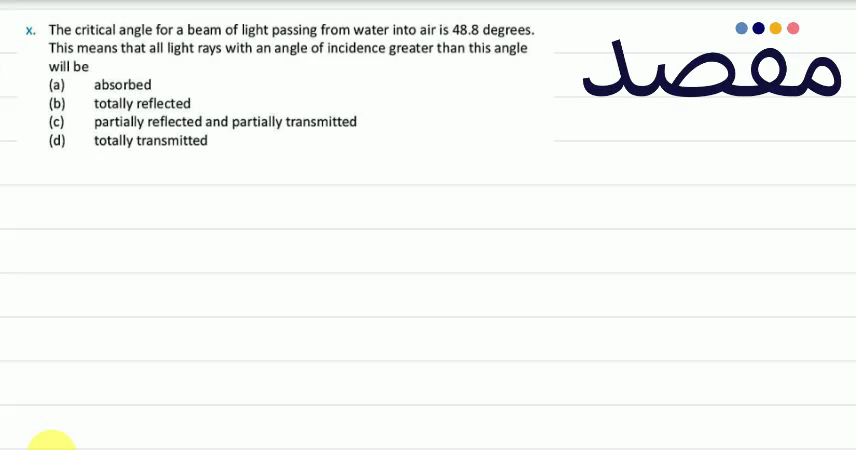# Classes

Change the way you learn with Maqsad's classes. Local examples, engaging animations, and instant video solutions keep you on your toes and make learning fun like never before!

Class 9Class 10First YearSecond Year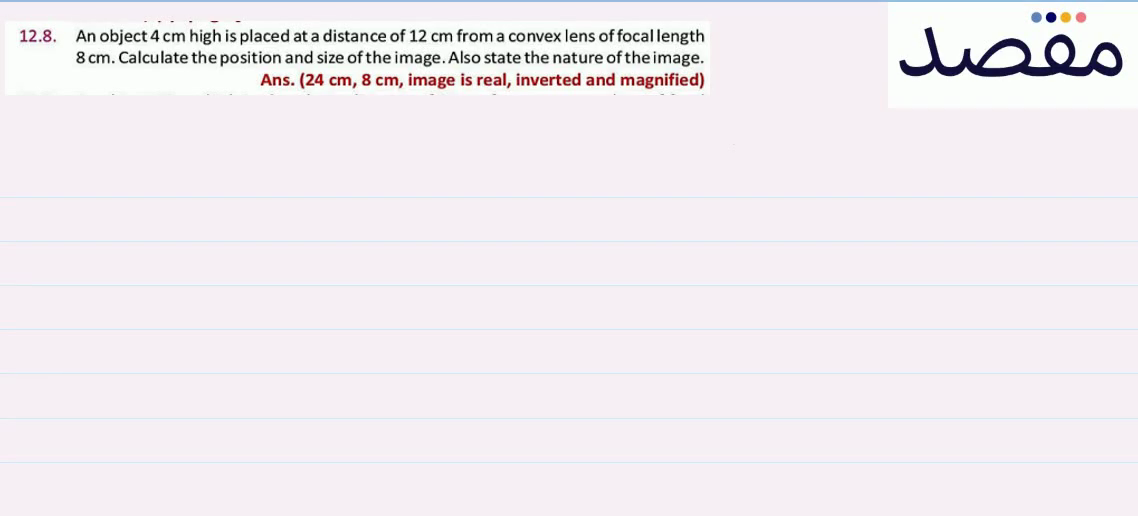### 12.8. An object 4 \mathrm{~cm} high is placed at a distance of 12 \mathrm{~cm} from a convex lens of focallength 8 \mathrm{~cm} . Calculate the position and size of the image. Also state the nature of the image.Ans. (24 \mathrm{cm} 8 \mathrm{~cm} image is real inverted and magnified)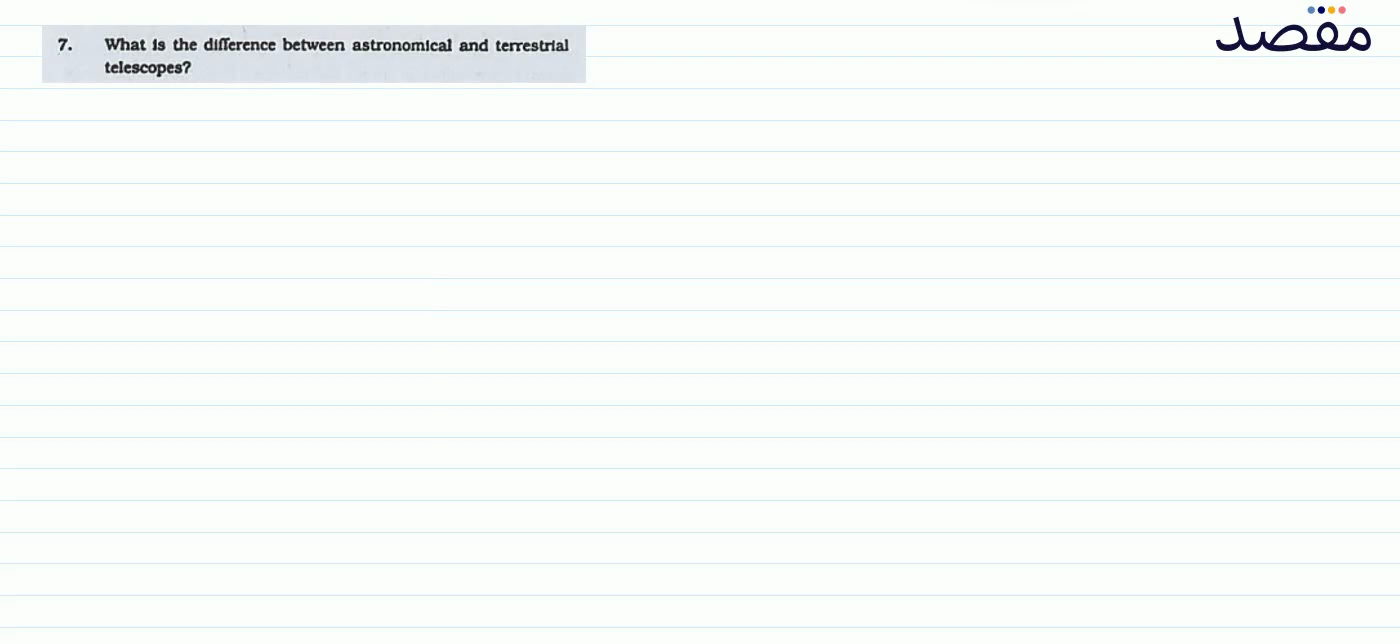### 7. What is the difference between astronomical and terrestrial telescopes?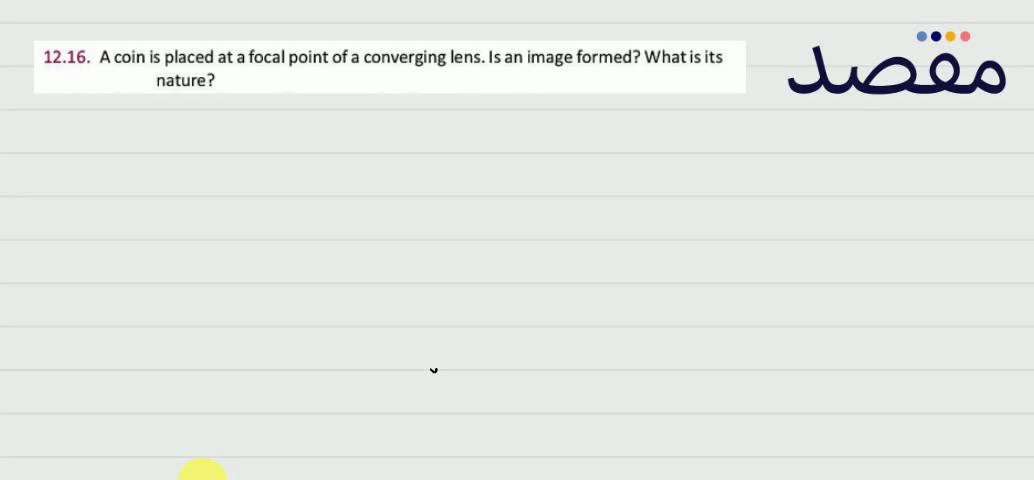### 12.16. A coin is placed at a focal point of a converging lens. Is an image formed? What is its nature?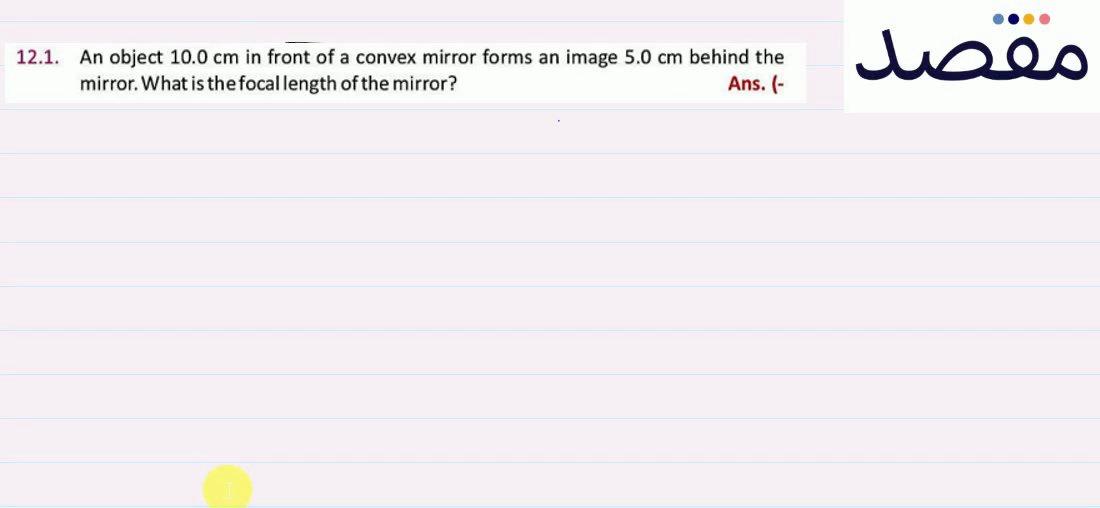### 12.1. An object 10.0 \mathrm{~cm} in front of a convex mirror forms an image 5.0 \mathrm{~cm} behind the mirror. What is the focal length of the mirror?Ans.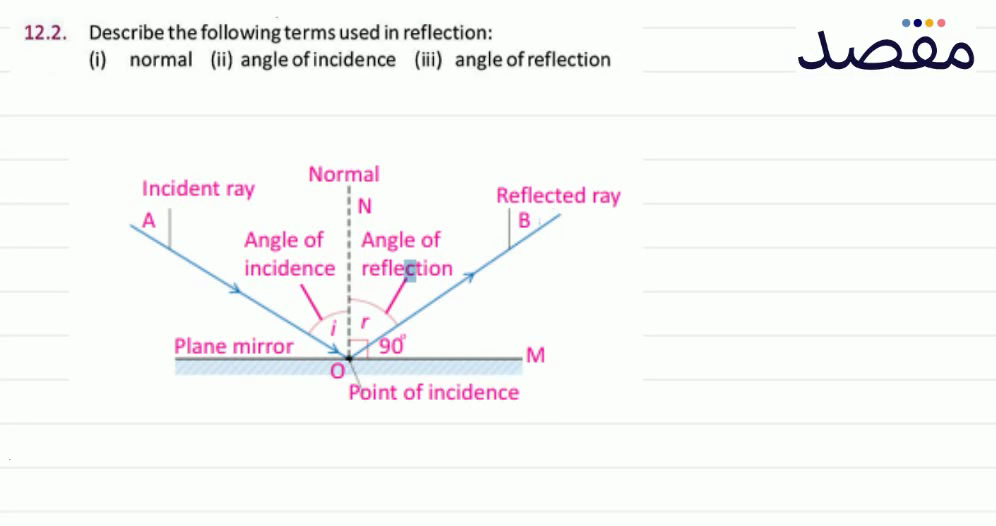### 12.2. Describe the following terms used in reflection:(i) normal (ii) angle of incidence (iii) angle of reflection